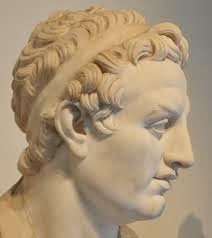# WHAT IS GEOGRAPHY?

 KALYANSIR.COM SHALL WE KNOW THE MEANING OF THE WORD GEOGRAPHY? ü  The word Geography has evolved from two Greek words. ü  Geo            -       The Earth ü  Graphein    -       To Write. ü  So Geography literally means “To write about the earth”. ü  In general term Geography is the study of the earth. ü  Hence we will be reading about the ·        Lithosphere – Solid earth crust ·        Atmosphere - Gases ·        Hydrosphere – Water  ü  Greek Scholars are considered to be the earliest Geographers.  ü  Hecate-us and Theophrastus are considered to be the earliest geographers.  ü  Kant defined Geography as the chorological science.  ü  The word Geography was first used by Eratosthenes (Greek scholar lived during 276–195 BC).  ü  Eratosthenes also credited for inventing the system of latitude and longitude. (These are the Horizontal and Vertical imaginary lines on the earth. We learn more about this in later chapters).  ü  Eratosthenes was also the first person to calculate the circumference of the earth.  ü  Eratosthenes was the first person to calculate the tilt of the Earth axis.

 Eratosthenes, one person credited with so many inventions. Isn't interesting? ·        The Word “Geography ·        Latitudes and Longitudes ·        Circumference of earth ·        Earth Axis ·        Leap Day

There are 3 important theories with reference to the earth.

1.      Geocentric Theory
2.      Heliocentric Theory
3.      Planetary Motion Theory

(Theory is an analysis given by a scientist. On single aspect, more than one scientist tries to explain his/her experiments/observations. Hence there are number of theories on various aspects).

Now let us try to know what are the theories that related to the earth?

1.      Geocentric Theory: (Earth is at the centre).

ü  This Theory is given by Ptolemy. (90 – 168 AD).PTOLEMY

ü  Ptolemy is the author of the book “Geography”.

ü  According to this theory the earth is at the centre of the Solar system.

 ü  Note: The Sun and its Planets put together is called the Solar system.
2.      Heliocentric Theory: (Sun is at the centre).

ü  This theory is given by Copernicus. (Poland, 1473 – 1543 AD).COPERNICUS

ü  According to this theory the Sun is at the centre of the Solar System.

3.      Planetary Motion Theory:

This theory is given by Kepler. (Germany, 1571 – 1630 AD).KEPLER

KALYANSIR.COM